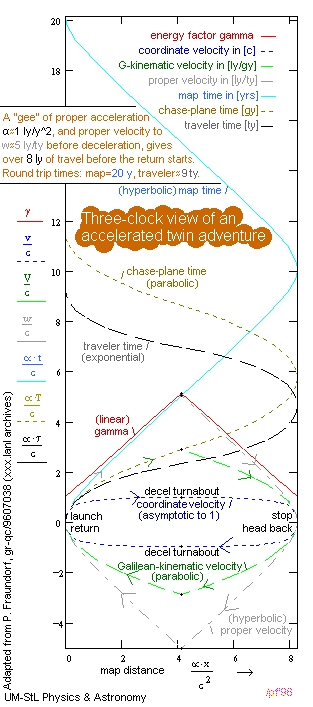# UM-StL Physics 112 - Andromeda Trip Followup

• Copyright (1970-95) by Phil Fraundorf
• Dept. of Physics & Astronomy, University of Missouri-StL, St. Louis MO 63121-4499
• Phone: (314)516-5044, Fax:(314)516-6152
• At UM-StLouis see also: accel1, cme, programs, stei-lab, & wuzzlers.
• A table of contents for these "frame-dependent relativity" pages.
• For source, cite URL at http://www.umsl.edu/~fraundor/rkinemat.html
• Mindquilts site page requests ~2000/day approaching a million per year.
• Requests for a "stat-counter linked subset of pages" since 4/7/2005: .
• Version release date: 18 July 1996.

## Cold ApplicationTest DeBrief

The revised draft of the Andromeda Trip provides a complete set of basic equations for solving relativistic 1D constant acceleration problems from the vantage point of a single inertial frame. The only prerequisite for this initiative is a familiarity with the "Galilean" constant acceleration equations commonly introduced in the first few chapters of all introductory physics texts.

The strategy is centered around the concept of a proper velocity w = dx/dτ, defined as the distance x traveled in our reference map frame per unit of traveler time τ elapsed. It is convenient to measure traveler-kinematic (proper) velocity in lightyears per traveler-year. This unit for proper velocity has been called a rodden-berry, as it is a "fundamental unit of hot rodding" which allows folk to appreciate the capability of super-colliders (e.g. a land speed record of 105 [rb] results in a head-on collision speed more like 1010 rb), while reminding folks that relativity increases rather than decreases the range of human travel possible as measured on the traveler's clock. In contrast, relativistic inertial coordinate velocity v is traditionally measured in units of one lightyear per coordinate-year, or c. There is no lightspeed limit on proper velocity, even though it is a property of spacetime (not any particular object's propagation) that coordinate velocity cannot exceed c. The strategy also builds on Galileo's classical equations for constant acceleration, which continue to work for "Galilean variables" V and T when extended into the relativistic regime by requiring that kinetic energy remain K = 0.5 mV^2. The caveat is that at high speeds supplementary equations are needed to predict the behavior of physical clocks, while Galilean time T becomes simply a mathematical parameter for keeping track of events.By measuring all distances in context of a single inertial reference frame, the concepts of Lorentz transform, length contraction, and frame-dependent simultaneity do not arise until after readers have a quantitative familiarity with the concepts of differential aging and relativistic acceleration. Another benefit of this strategy is our ability to use x-tv diagrams for plotting the value of everyone's time and velocity variables as a function of object position on a single graph, as illustrated in the twin adventure plot here. Note: Assuming that acceleration ao is approximately 1 "gee" on this plot, then distances are in [lightyears], times are in [years], and velocities are in [lightyears per year].

## More Problems

Try the Andromeda Problem challenge for a trip of 4 lightyears to the nearest star, alpha-Centauri, instead of 2 million lightyears to the nearest galaxy. Since the exponential "e-multiplying time c/a" is about a year, for distance-traveled with 1 gee acceleration, the effects may not be as large as they are for the longer journey.

## Solutions

Hint: If you could keep that 1 gee acceleration going for much less than a human lifetime on the proposed trip, you would find yourself 2 million lightyears away and 2 million years in the future! If you want to see a full numerical solution to the Andromeda problem, check here.
Send comments, your answers to problems posed, and/or complaints, to philf@newton.umsl.edu. This page contains original material, so if you choose to echo in your work, in print, or on the web, a citation would be cool. ` (Thanks. /philf :)`